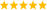# LabVIEW

cancel
Showing results for
Did you mean:

Highlighted

## Computing the value of sin(x)

I have a lab that I have been working on and just cannot figure out. If anyone had any input it would be greatly appreciated.

The lab is to: Develop a VI that computes the value of sin(x) at a given x using n terms of the series expansion of the sine function.

-Create a control which represents the value of n in the sin(x) equation

-Create an indicator to show the result of sin(x)

I have been playing around with loops and cannot figure it out.

Thanks

Message 1 of 26
(3,143 Views)
Highlighted

## Re: Computing the value of sin(x)

The series expansion is a number of x-exponents and factors, right? According to your instruction you should use N of those.

So, make a list of the exponents and factors (actually you only need the factors), loop through them with N as loop counter and x^i * factor, sum up the result.

/Y

"Only dead fish swim downstream" - "My life for Kudos!" - "Dumb people repeat old mistakes - smart ones create new ones."Message 2 of 26
(3,135 Views)
Highlighted

## Re: Computing the value of sin(x)

Also, post the code you have. We will guide you, but not do the work entirely. 😉

Putnam
Certified LabVIEW Developer

Senior Test Engineer North Shore Technology, Inc.
Currently using LV 2012-LabVIEW 2018, RT8.5LabVIEW Champion

Message 3 of 26
(3,122 Views)
Highlighted

## Re: Computing the value of sin(x)

As a first step, find the taylor series expansion for sin(x). Do you have that?

@waltonj1 wrote:

I have been playing around with loops and cannot figure it out.

All you probably need is a single loop, not loops. Show us what you have and we can probably point you in the right direction.

(If you would have searched the forum, you probably would have found some interesting discussions, such as this one.)LabVIEW Champion. It all comes together inGCentralWhat does "Engineering Redefined" mean??
Message 4 of 26
(3,101 Views)
Highlighted

## Re: Computing the value of sin(x)

I read that but it did not help too much. Heres the series expansion$\sin x = \sum^{\infty}_{n=0} \frac{(-1)^n}{(2n+1)!} x^{2n+1} = x - \frac{x^3}{3!} + \frac{x^5}{5!} - \cdots\quad\text{ for all } x\!$

Here is the problem (#2)Here's what I have now. Please ignore the formula I have in the loop, I believe it is wrong...Message 5 of 26
(3,088 Views)
Highlighted

## Re: Computing the value of sin(x)

I see that the proper capitalization of LabVIEW is not being taught these days....Message 6 of 26
(3,083 Views)
Highlighted

## Re: Computing the value of sin(x)

I always do the proper capitlization myself! You can see by the name of the file 🙂

Message 7 of 26
(3,077 Views)
Highlighted

## Re: Computing the value of sin(x)

@waltonj1 wrote:

I read that but it did not help too much.

What is "that"?LabVIEW Champion. It all comes together inGCentralWhat does "Engineering Redefined" mean??
Message 8 of 26
(3,068 Views)
Highlighted

Message 9 of 26
(3,065 Views)
Highlighted

## Re: Computing the value of sin(x)

How exactly do I go about that? Sorry...new

Thanks

Message 10 of 26
(3,061 Views)